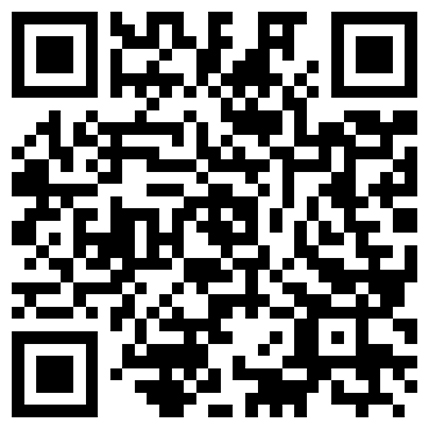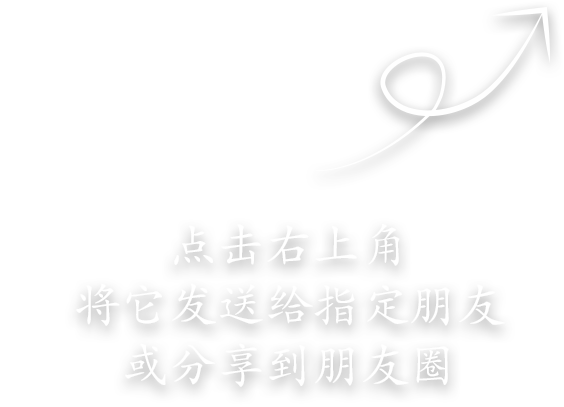• 手机版

用手机扫一扫

• 会员中心you postion

# Kirchhoff's first law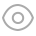131

Kirchhoff's current law is also known as Kirchhoff's first law (KCL for short). It is a node used in a circuit. The so-called node refers to the point where three or more branches of the circuit are connected.

Kirchhoff current law points out that: for any node in the circuit, at any time, the current flowing into the node is equal to the current flowing out of the node; That is, the algebraic sum of node currents is equal to 0 (constant means forever). It is expressed by mathematical formula as:

Kirchhoff's current law

The above formula shows that at the junction point of current, the algebraic sum of current is equal to zero. The reason why it is equal to zero is that it is customary to specify that the current flowing into the node is positive and the current flowing out of the node is negative.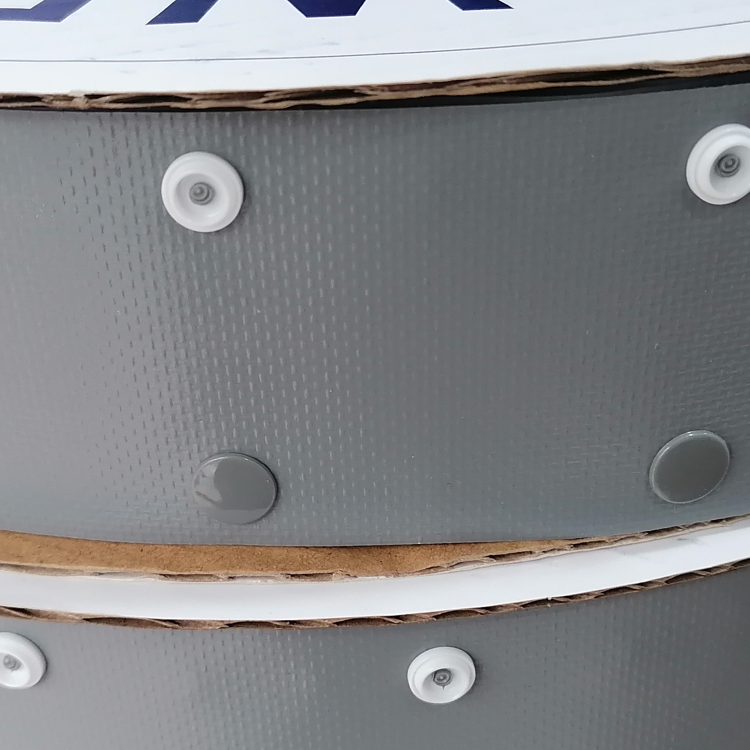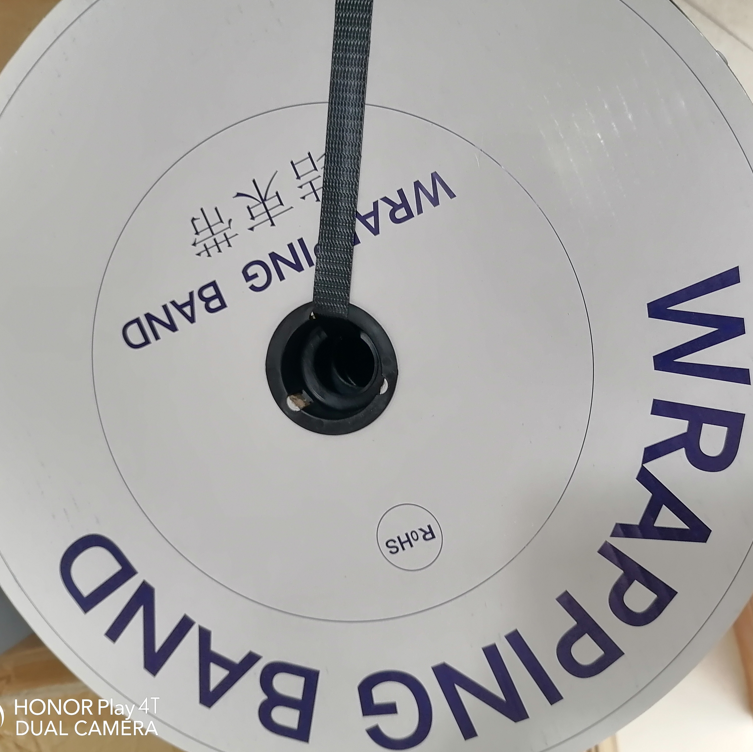Theso

Shanghai Fakong Industrial and Trading Co.,Ltd.